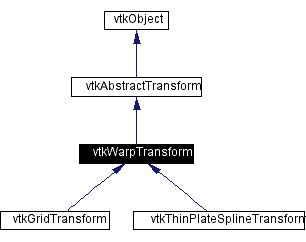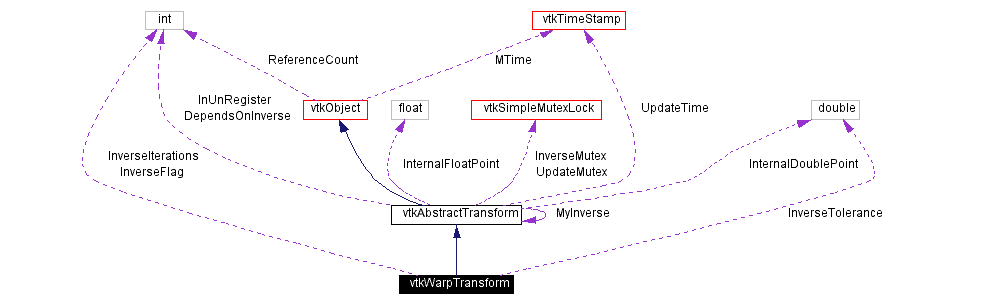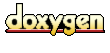Main Page   Class Hierarchy   Alphabetical List   Compound List   File List   Compound Members   File Members   Related Pages

# vtkWarpTransform Class Reference

superclass for nonlinear geometric transformations. More...

`#include <vtkWarpTransform.h>`

Inheritance diagram for vtkWarpTransform:[legend]
Collaboration diagram for vtkWarpTransform:[legend]
List of all members.

## Public Methods

virtual const char * GetClassName ()
virtual int IsA (const char *type)
void PrintSelf (ostream &os, vtkIndent indent)
void Inverse ()
virtual void SetInverseTolerance (double)
virtual double GetInverseTolerance ()
virtual void SetInverseIterations (int)
virtual int GetInverseIterations ()
void InternalTransformPoint (const float in, float out)
void InternalTransformPoint (const double in, double out)
void InternalTransformDerivative (const float in, float out, float derivative)
void InternalTransformDerivative (const double in, double out, double derivative)
void TemplateTransformPoint (const float in, float out)
void TemplateTransformPoint (const double in, double out)
void TemplateTransformPoint (const float in, float out, float derivative)
void TemplateTransformPoint (const double in, double out, double derivative)
void TemplateTransformInverse (const float in, float out)
void TemplateTransformInverse (const double in, double out)
void TemplateTransformInverse (const float in, float out, float derivative)
void TemplateTransformInverse (const double in, double out, double derivative)

## Static Public Methods

int IsTypeOf (const char *type)
vtkWarpTransform * SafeDownCast (vtkObject *o)

## Protected Methods

vtkWarpTransform ()
~vtkWarpTransform ()
vtkWarpTransform (const vtkWarpTransform &)
void operator= (const vtkWarpTransform &)
virtual void ForwardTransformPoint (const float in, float out)=0
virtual void ForwardTransformPoint (const double in, double out)=0
virtual void ForwardTransformDerivative (const float in, float out, float derivative)=0
virtual void ForwardTransformDerivative (const double in, double out, double derivative)=0
virtual void InverseTransformPoint (const float in, float out)
virtual void InverseTransformPoint (const double in, double out)
virtual void InverseTransformDerivative (const float in, float out, float derivative)
virtual void InverseTransformDerivative (const double in, double out, double derivative)

## Protected Attributes

int InverseFlag
int InverseIterations
double InverseTolerance

## Detailed Description

superclass for nonlinear geometric transformations.

Date:
2000/12/10 20:08:21
Revision:
1.2
Thanks:
Thanks to David G. Gobbi who developed this class.
vtkWarpTransform provides a generic interface for nonlinear warp transformations.
vtkThinPlateSplineTransform vtkGridTransform vtkGeneralTransform

Definition at line 57 of file vtkWarpTransform.h.

## Constructor & Destructor Documentation

 vtkWarpTransform::vtkWarpTransform ( ) ` [protected]`

 vtkWarpTransform::~vtkWarpTransform ( ) ` [protected]`

 vtkWarpTransform::vtkWarpTransform ( const vtkWarpTransform & ) ` [inline, protected]`
 Definition at line 123 of file vtkWarpTransform.h.

## Member Function Documentation

 virtual const char* vtkWarpTransform::GetClassName ( ) ` [virtual]`
 Return the class name as a string. This method is defined in all subclasses of vtkObject with the vtkTypeMacro found in vtkSetGet.h. Reimplemented from vtkAbstractTransform. Reimplemented in vtkGridTransform, and vtkThinPlateSplineTransform.

 int vtkWarpTransform::IsTypeOf ( const char * type ) ` [static]`
 Return 1 if this class type is the same type of (or a subclass of) the named class. Returns 0 otherwise. This method works in combination with vtkTypeMacro found in vtkSetGet.h. Reimplemented from vtkAbstractTransform. Reimplemented in vtkGridTransform, and vtkThinPlateSplineTransform.

 virtual int vtkWarpTransform::IsA ( const char * type ) ` [virtual]`
 Return 1 if this class is the same type of (or a subclass of) the named class. Returns 0 otherwise. This method works in combination with vtkTypeMacro found in vtkSetGet.h. Reimplemented from vtkAbstractTransform. Reimplemented in vtkGridTransform, and vtkThinPlateSplineTransform.

 vtkWarpTransform* vtkWarpTransform::SafeDownCast ( vtkObject * o ) ` [static]`
 Will cast the supplied object to vtkObject* is this is a safe operation (i.e., a safe downcast); otherwise NULL is returned. This method is defined in all subclasses of vtkObject with the vtkTypeMacro found in vtkSetGet.h. Reimplemented from vtkAbstractTransform. Reimplemented in vtkGridTransform, and vtkThinPlateSplineTransform.

 void vtkWarpTransform::PrintSelf ( ostream & os, vtkIndent indent ) ` [virtual]`
 Methods invoked by print to print information about the object including superclasses. Typically not called by the user (use Print() instead) but used in the hierarchical print process to combine the output of several classes. Reimplemented from vtkAbstractTransform. Reimplemented in vtkGridTransform, and vtkThinPlateSplineTransform.

 void vtkWarpTransform::Inverse ( ) ` [virtual]`
 Invert the transformation. Warp transformations are usually inverted using an iterative technique such as Newton's method. The inverse transform is usually around five or six times as computationally expensive as the forward transform. Reimplemented from vtkAbstractTransform.

 virtual void vtkWarpTransform::SetInverseTolerance ( double ) ` [virtual]`
 Set the tolerance for inverse transformation. The default is 0.001.

 virtual double vtkWarpTransform::GetInverseTolerance ( ) ` [virtual]`

 virtual void vtkWarpTransform::SetInverseIterations ( int ) ` [virtual]`
 Set the maximum number of iterations for the inverse transformation. The default is 500, but usually only 2 to 5 iterations are used. The inversion method is fairly robust, and it should converge for nearly all smooth transformations that do not fold back on themselves.

 virtual int vtkWarpTransform::GetInverseIterations ( ) ` [virtual]`

 void vtkWarpTransform::InternalTransformPoint ( const float in, float out ) ` [virtual]`
 This will calculate the transformation without calling Update. Meant for use only within other VTK classes. Reimplemented from vtkAbstractTransform.

 void vtkWarpTransform::InternalTransformPoint ( const double in, double out ) ` [virtual]`
 Reimplemented from vtkAbstractTransform.

 void vtkWarpTransform::InternalTransformDerivative ( const float in, float out, float derivative ) ` [virtual]`
 This will calculate the transformation, as well as its derivative without calling Update. Meant for use only within other VTK classes. Reimplemented from vtkAbstractTransform.

 void vtkWarpTransform::InternalTransformDerivative ( const double in, double out, double derivative ) ` [virtual]`
 Reimplemented from vtkAbstractTransform.

 void vtkWarpTransform::TemplateTransformPoint ( const float in, float out ) ` [inline]`
 Do not use these methods. They exists only as a work-around for internal templated functions (I really didn't want to make the Forward/Inverse methods public, is there a decent work around for this sort of thing?) Definition at line 98 of file vtkWarpTransform.h.

 void vtkWarpTransform::TemplateTransformPoint ( const double in, double out ) ` [inline]`
 Definition at line 100 of file vtkWarpTransform.h.

 void vtkWarpTransform::TemplateTransformPoint ( const float in, float out, float derivative ) ` [inline]`
 Definition at line 102 of file vtkWarpTransform.h.

 void vtkWarpTransform::TemplateTransformPoint ( const double in, double out, double derivative ) ` [inline]`
 Definition at line 105 of file vtkWarpTransform.h.

 void vtkWarpTransform::TemplateTransformInverse ( const float in, float out ) ` [inline]`
 Definition at line 108 of file vtkWarpTransform.h.

 void vtkWarpTransform::TemplateTransformInverse ( const double in, double out ) ` [inline]`
 Definition at line 110 of file vtkWarpTransform.h.

 void vtkWarpTransform::TemplateTransformInverse ( const float in, float out, float derivative ) ` [inline]`
 Definition at line 112 of file vtkWarpTransform.h.

 void vtkWarpTransform::TemplateTransformInverse ( const double in, double out, double derivative ) ` [inline]`
 Definition at line 115 of file vtkWarpTransform.h.

 void vtkWarpTransform::operator= ( const vtkWarpTransform & ) ` [inline, protected]`
 Definition at line 124 of file vtkWarpTransform.h.

 virtual void vtkWarpTransform::ForwardTransformPoint ( const float in, float out ) ` [protected, pure virtual]`
 If the InverseFlag is set to 0, then a call to InternalTransformPoint results in a call to ForwardTransformPoint. Reimplemented in vtkGridTransform, and vtkThinPlateSplineTransform.

 virtual void vtkWarpTransform::ForwardTransformPoint ( const double in, double out ) ` [protected, pure virtual]`
 Reimplemented in vtkGridTransform, and vtkThinPlateSplineTransform.

 virtual void vtkWarpTransform::ForwardTransformDerivative ( const float in, float out, float derivative ) ` [protected, pure virtual]`
 Calculate the forward transform as well as the derivative. Reimplemented in vtkGridTransform, and vtkThinPlateSplineTransform.

 virtual void vtkWarpTransform::ForwardTransformDerivative ( const double in, double out, double derivative ) ` [protected, pure virtual]`
 Reimplemented in vtkGridTransform, and vtkThinPlateSplineTransform.

 virtual void vtkWarpTransform::InverseTransformPoint ( const float in, float out ) ` [protected, virtual]`
 If the InverseFlag is set to 1, then a call to InternalTransformPoint results in a call to InverseTransformPoint. The inverse transformation is calculated from using Newton's method. Reimplemented in vtkGridTransform.

 virtual void vtkWarpTransform::InverseTransformPoint ( const double in, double out ) ` [protected, virtual]`
 Reimplemented in vtkGridTransform.

 virtual void vtkWarpTransform::InverseTransformDerivative ( const float in, float out, float derivative ) ` [protected, virtual]`
 Calculate the inverse transform as well as the derivative of the forward transform (that's correct: the derivative of the forward transform, not of the inverse transform) Reimplemented in vtkGridTransform.

 virtual void vtkWarpTransform::InverseTransformDerivative ( const double in, double out, double derivative ) ` [protected, virtual]`
 Reimplemented in vtkGridTransform.

## Member Data Documentation

 int vtkWarpTransform::InverseFlag` [protected]`
 Definition at line 151 of file vtkWarpTransform.h.

 int vtkWarpTransform::InverseIterations` [protected]`
 Definition at line 152 of file vtkWarpTransform.h.

 double vtkWarpTransform::InverseTolerance` [protected]`
 Definition at line 153 of file vtkWarpTransform.h.

The documentation for this class was generated from the following file:

Generated on Wed Nov 21 13:02:44 2001 for VTK by1.2.11.1 written by Dimitri van Heesch, © 1997-2001